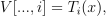/NumPy 1.17

# numpy.polynomial.chebyshev.chebvander

`numpy.polynomial.chebyshev.chebvander(x, deg)` [source]

Pseudo-Vandermonde matrix of given degree.

Returns the pseudo-Vandermonde matrix of degree `deg` and sample points `x`. The pseudo-Vandermonde matrix is defined bywhere `0 <= i <= deg`. The leading indices of `V` index the elements of `x` and the last index is the degree of the Chebyshev polynomial.

If `c` is a 1-D array of coefficients of length `n + 1` and `V` is the matrix `V = chebvander(x, n)`, then `np.dot(V, c)` and `chebval(x, c)` are the same up to roundoff. This equivalence is useful both for least squares fitting and for the evaluation of a large number of Chebyshev series of the same degree and sample points.

Parameters: `x : array_like` Array of points. The dtype is converted to float64 or complex128 depending on whether any of the elements are complex. If `x` is scalar it is converted to a 1-D array. `deg : int` Degree of the resulting matrix. `vander : ndarray` The pseudo Vandermonde matrix. The shape of the returned matrix is `x.shape + (deg + 1,)`, where The last index is the degree of the corresponding Chebyshev polynomial. The dtype will be the same as the converted `x`.

© 2005–2019 NumPy Developers
Licensed under the 3-clause BSD License.
https://docs.scipy.org/doc/numpy-1.17.0/reference/generated/numpy.polynomial.chebyshev.chebvander.html